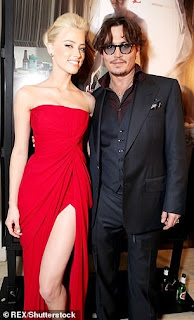## Friday, 4 September 2020

### Johnny Depp - Profit Margin & Mark Up

PROFIT MARGIN & PROFIT MARK UPJohnny Depp married Amber Heard in February 2015, and she filed for divorce in May 2016, which was finalized in January 2017 and Depp paid a settlement of \$7m.

In 2019, Depp sued Heard for \$50m!!!

What level of profit margin is this???? LOVE GONE DEAD!!!

Profit margin

This is gross profit expressed as a percentage of the selling price. It is a percentage profit charged on the sales. So sales represents 100%; where profit margin is 20%; sales will represent 100% and cost will represent 80%.

Gross profit    x 100

Selling price

Profit mark-up

This is gross profit expressed as a percentage of the cost of goods which is added to the cost price to arrive at the selling price. So sales will represent more than 100%; where profit mark-up is 20%, sales will represent 120% and cost will represent 100%.

Gross profit    x 100

Cost price

The mark-up is a fraction of the cost price, while margin is a fraction of the selling price. Using profit margin, the selling price/ sales will always be represented at 100%, but with mark-up the selling price/ sales is represented at above 100% by the mark-up %.

COST + PROFIT = SALES

Where a business makes 20% margin, it can be expressed as below;

PROFIT MARGIN = 80% COST + 20% PROFIT = 100% SALES

Where a business makes 20% mark-up, it can be expressed as below;

PROFIT MARK-UP = 100% COST + 20% PROFIT = 120% SALES

So, for Johnny Depp’s position;

Cost price = \$7m

Sales price = \$50m

Profit = \$(50-7)m = \$43m

With profit margin, it will mean that the sales price is 100% and the profit margin is 43/50x100= 86%

With profit mark-up, it will mean that the cost is 100% and the profit mark-up is 43/7x100= 614%

The above two calculations show profit being expressed as a percentage of sales (profit margin) and cost (profit mark-up).